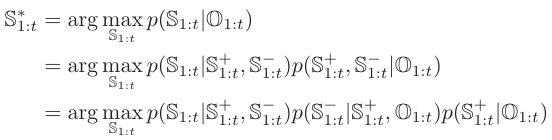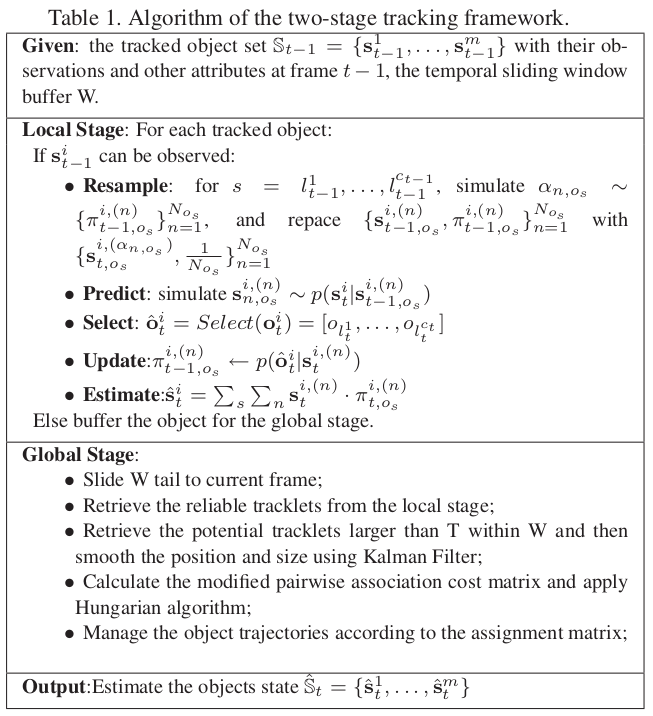# Local Tracklets Filtering and Global Tracklets Association

##### Posted on Jul 05, 2021 (Update: Jul 06, 2021) 0 Comments

The accuracy of state-of-the-art object detectors is still far from perfect. The detection performance is usually a tradeoff between the detection rate and the false alarm rate.

• missed detections and false alarms and inaccurate responses happen frequently in the detection procedure which provides misleading information to tracking algorithms.
• detection-based tracking method must overcome these failures of the detector, and the difficulties caused by occlusions and similar appearance among multiple objects

the paper aims to

• overcome the limitations of current object detectors to track multiple objects through occlusions online in dense surveillance scenarios.
• propose an online detection-based two-stage multi-object tracking method.
• in the local stage, use a particle filter to generate a set of reliable tracklets
• in the global stage, collect the detection responses from a temporal sliding window to generate a set of potential tracklets
• the reliable tracklets generated in the local stage and the potential tracklets generated within the temporal sliding window are associated by Hungarian algorithm.

Given detection responses generated by the detector, the tracking method needs to retrieve the real objects among these responses and set ID for each of them in every frame. Two strategies to deal with the data association problem,

1. associate the response locally (frame by frame)
2. associate them globally
• Wu et al.: define an affinity measure between detection responses based on cues from position, size, and color and used a greedy algorithm to associate object hypotheses and detection responses.
• Li et al. and Okuma et al.: use particle methods to associate the detection responses of an unknown number of objects. The detection responses were used to generate new particles and evaluate existing particles.
• Li et al.: use multiple detectors (observers) to form a cascade particle filter.
• the local association methods used only the information in two consecutive frames which makes them incline to drift (??) when multiple objects are close to each other.

To overcome the drifting problem of local association method,

• one approach is to optimize multiple trajectories simultaneously such as in Multi-Hypothesis Tracking or in Joint Probabilistic Data Association Filters (JPDAF)
• Leibe et al.: use Quadratic Boolean Programming to couple the detection and estimation of trajectory hypotheses
• Andriluka et al.: use Viterbi algorithm to get optimal object sequences
• Zhang et al.: use a cost-flow network to model the MAP data association problem
• Huang et al.: did data association of detection responses hierarchically.

Let $s_t^i=(p_t^i, s_t^i)$ be the state of a particular object $i$ at frame $t$

• $s_{1:t}^i={s_1^i,\ldots,s_t^i}$: the trajectory of the object $i$ up to frame $t$
• $S_t={s_t^1,\ldots,s_t^m}$: all the object states appeared at frame $t$
• $S_{1:t}={s_{1:t}^1,\ldots, s_{1:t}^m}$: all object trajectories up to frame $t$

observations are generated by applying a detector on each cell video frame.

• $o_t^i$: the $i$-th object collected at frame $t$ given the object state $s_t^i$
• $o_{1:t}^i = {o_1^i,\ldots, o_t^i}$: all the observations of object $i$ up to frame $t$. (Is it known? Already know which is object $i$?)
• $O_t={o_t^1,\ldots,o_t^m}$: all the observations collected at frame $t$
• $O_{1:t}={o_{1:t}^1,\ldots,o_{1:t}^m}$: all the observations up to frame $t$

The final aim is to find the optimal trajectories for all the objects based on the observation set. It is equivalent to maximize the posteriori probability of $S_{1:t}$ giving the observations $O_{1:t}$:

$S_{1:t}^\star = \argmax_{S_{1:t}}p(S_{1:t}\mid O_{1:t})$

confused…

Since the number of all possible enumerations of $S_{1:t}$ given $O_{1:t}$ is huge, it prevents a brute force search to find the global optimum.

1. optimize locally on each object which is equivalent to maximize the posterior probability of $s_t^i$ giving the observation $o_{1:t}^i$ (the observations of $i$ have already known???):
$s_t^{\star i}=\argmax_{s_t^i}p(s_t^i\mid o_{1:t}^i)\,.$

this could be done by a particle filter, and denote the tracklet set generated as $S_{1:t}^+$.

1. around trajectory break points of potential tracklets detection responses from a temporal sliding window are generated and associated by greedy linking method. Denote this tracklet set generated as $S_{1:t}^-$.

2. data association will be done on ${S_{1:t}^+, S_{1:t}^-}$three types of occlusion due to the causation:

• object self-occlusion
• inter-objects occlusion
• object occlusion by other scene objects

two types:

• partial occlusion: the paper uses a multi-view multi-part human detector to collect observations and a particular filter with observer selection process to deal with partial object occlusion
• full occlusion: track it by data association of the detection responses within a temporal sliding window

partition the human body into three levels

• full-body

A human hypothesis consists of three overlapped part areas of the human body. Denote the detection response as a 6-tuple $rp = {l, p, s, t, v, a}$, where

• $l$: the label indicating the response type which could be FB, HS or HT
• $p$: the position
• $s$: the size
• $t$: frame index
• $v$: the visible score
• $a$: the appearance model

A combined response: $rc = {rp_i\mid l_i=FB, HS, HT}$

A human hypothesis $H$ can be represented as ${rc, u}$ where $u$ indicates the visible part. - if $u$ is none of three response types, it means the human is not visible

## local tracklets filtering

if the object state can not be observed (all the parts are invisible or lost by the observation model), the local tracklet filtering stage on this object stops and the tracklet is buffered for the global stage.

still confused how the local tracklets can assign the observations for the same object when updating

## global tracklets association

detection responses are associated within a temporal sliding window around trajectory break points (tails of reliable tracklets)

### detection response association for potential tracklets

at each frame within the temporal sliding window,

1. collect object hypotheses according to detection responses
2. add occulded object hypotheses according to the tracking results at the local stage (?????). These object hypotheses are then associated with the object hypotheses in the previous frame based on the affinity of the responses in position, size and appearance
3. if an object hypothesis is associated in T consecutive frames, then generate a new potential tracklet

### pairwise tracklet association for final object trajectories

suppose there are $m$ tracklets in the Reliable Tracklets set $S_{1:t}^+$ and $n$ tracklets in the Potential Tracklets set $S_{1:t}^-$.

four situations:

• $TR_i$ -> $TP_j$
• $TR_i$ is a terminal
• $TP_j$ is an initial
• $TP_j$ is a false trajectory

describe a tracklet $T_i$ by a 3-tuple ${A_i,S_i,M_i}$, where

• $A_i$ is the appearance model
• $S_i$ is the shape model
• $M_i$ is the motion model

calculate both the forward velocity and backward velocity of the tracklet as its motion model.my understanding:

in the local stage, the observers know the location of the object, as said in

When the system has a reliable observation model, the state of the object can be well updated. This corresponds to the situation when one object is isolated from other objects and the detector can generate good observations.

sometimes it might not be accurate enough,

But in the dense environment where multiple objects are close to each other and not all of the human bodies are fully visible, the detector will fail to generate reliable observations or even give wrong responses. … …propose to select the best subset of corresponding observations in the particle filter procedure. … the select procedure selects the best subset $\hat o_t = (…)$.

so is it true to say a state has multiple observation candidates?

## Conclusions

present an online detection-based two-stage multi-object tracking framework which seeks both the local optimum trajectory for each object

is it true that the local stage does not involve any association, and the observer can directly observe the object?

and the global optimum trajectories for all tracked objects

it integrates particle filter based tracker with data association based tracker efficiently to guarantee online tracking performance.

• different from the classical particle filter which results in many breaks of object trajectories due to occlusions
• different from the conventional association based tracker which is time consuming and usually offline.

Published in categories Note